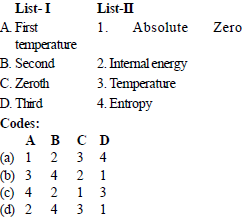# Test: Thermodynamic (Level - II)- 2

## 25 Questions MCQ Test Mechanical Engineering SSC JE (Technical) | Test: Thermodynamic (Level - II)- 2

Description
This mock test of Test: Thermodynamic (Level - II)- 2 for Mechanical Engineering helps you for every Mechanical Engineering entrance exam. This contains 25 Multiple Choice Questions for Mechanical Engineering Test: Thermodynamic (Level - II)- 2 (mcq) to study with solutions a complete question bank. The solved questions answers in this Test: Thermodynamic (Level - II)- 2 quiz give you a good mix of easy questions and tough questions. Mechanical Engineering students definitely take this Test: Thermodynamic (Level - II)- 2 exercise for a better result in the exam. You can find other Test: Thermodynamic (Level - II)- 2 extra questions, long questions & short questions for Mechanical Engineering on EduRev as well by searching above.
QUESTION: 1

Solution:
QUESTION: 2

Solution:
QUESTION: 3

### The term m Dh in a control volume equation Q – W = mDh

Solution:
QUESTION: 4

Select the Kelvin-Plank statement of the second law:

Solution:
QUESTION: 5

According to the Clausiu’s statement of the second law:
1. heat flows from cold surface to hot surface, unaided.
2. heat flows from hot surface to cold surface, unaided.
3. heat can flow from cold surface to hot surface with the aid of external work.
Which of the above statement is /are correct ?

Solution:
QUESTION: 6

The more effective way of increasing the efficiency of a carnot engine is to

Solution:
QUESTION: 7

Consider the following statements:
1. It is the second law of thermodynamics which provides criterion as to the probability of various processes.
2. Heat and work are completely interchangeable forms of energy.
3. Work is high grade energy.
4. The complete conversion of low grade energy into high grade energy in a cycle is impossible
Which of the above statements are correct ?

Solution:
QUESTION: 8

The four processes of the carnot cycle are

Solution:
QUESTION: 9

Perpetual motion machine of second kind violates the

Solution:
QUESTION: 10

The efficiency of the carnot cycle may be increased by

Solution:
QUESTION: 11

The unit of energy in S.I. units is

Solution:
QUESTION: 12

An engine operates between temperature limits of 900 K and T2 and another between T2 and 400 K for both to be equally efficient T2 will be equal to

Solution:
QUESTION: 13

For the same above engine, if work output is to be equal than T2 will be equal to

Solution:
QUESTION: 14

Three engines A, B and C operating on carnot cycle respectively use air, steam and Helium as the working fluid. If all the engine operates within the same temperature limit, then which engine will have highest efficiency

Solution:
QUESTION: 15

If a heat engine gives an output of 3 kW when the input is 10000 J/sec then thermal efficiency of the engine will be

Solution:
QUESTION: 16

The pressure’of a gas in terms of its mean kinetic energy per unit volume E is equal to

Solution:
QUESTION: 17

Match List - I ( Laws of thermodynamics) with List -II (Defines) the following:Solution:
QUESTION: 18

The slope of constant pressure line on a T-S diagram is given by

Solution:
QUESTION: 19

In statistical thermodynamics, entropy is defined as
1. Measure of irreversibility of a system
2. A universal property
3. Degree of randomness
4. Thermodynamic probability of disorderness
Which of the above is/are correct ?

Solution:
QUESTION: 20

Consider the following statements: In an irreversible process
1. entropy always increases
2. the sum of the entropy of all bodies taking part in a process always increases
3. Once created, entropy can not be destroyed
Which among the above is /are correct ?

Solution:
QUESTION: 21

One kg of water at room temperature is brought into contact with a high temperature thermal reservoir. The entropy change of the universe is

Solution:
QUESTION: 22

For a thermodynamics cycle to be irreversible, it is necessary that

Solution:
QUESTION: 23

In a reversible cycle, the entropy of the system

Solution:
QUESTION: 24

The entropy change for any closed system which undergoes an irreversible adiabatic process

Solution:
QUESTION: 25

Consider two subsystem 1 and 2 containing same fluid and having same mass m; but at Temperature T1 and T2 (T1 > T2) enclosed in an adiabatic enclosure separate by a partition. If the partition is removed and the fluids are allowed to mix. The entropy change of process is

Solution: